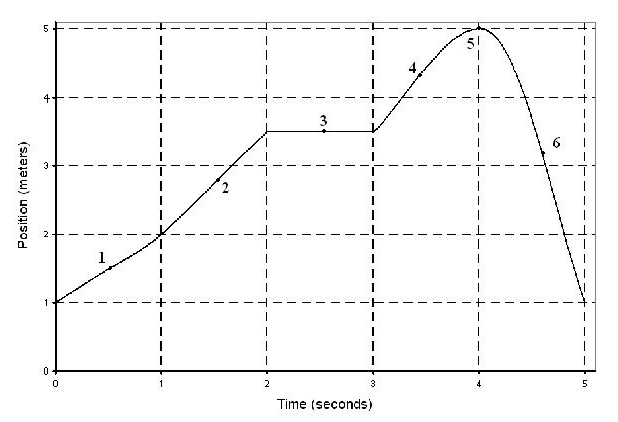# Problem: Questions go with graph:1.  Its speed at point 1 is less than the speed at point 4. true or false?2. Where is its x-component of velocity zero for only a moment? A. 5 B. 6 C. 1 D. 2 E. 3 F. 4

###### FREE Expert Solution

1.

The slope of a position-time graph gives the speed of the particle.

96% (225 ratings)###### Problem Details

Questions go with graph:1.  Its speed at point 1 is less than the speed at point 4. true or false?

2. Where is its x-component of velocity zero for only a moment?

A. 5

B. 6

C. 1

D. 2

E. 3

F. 4